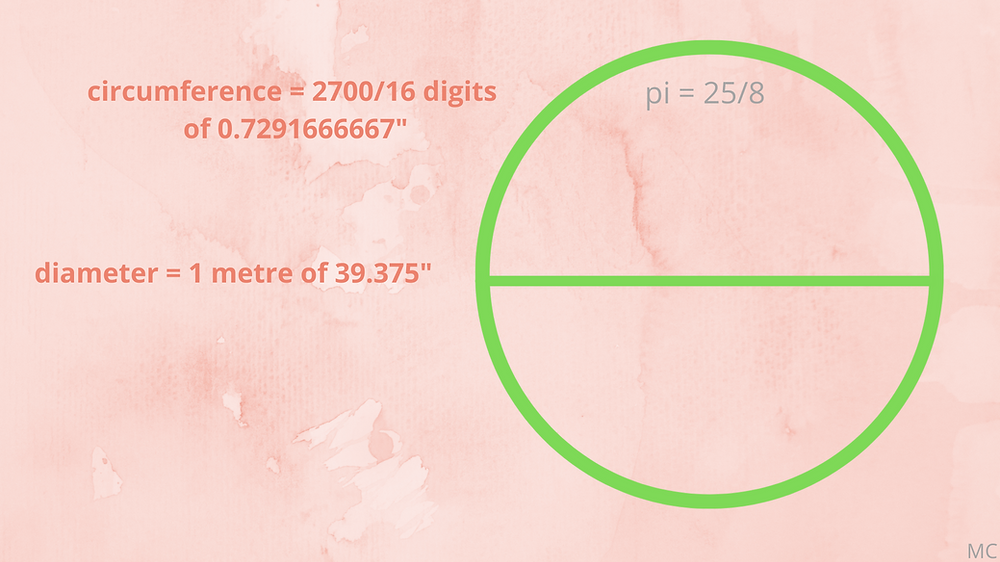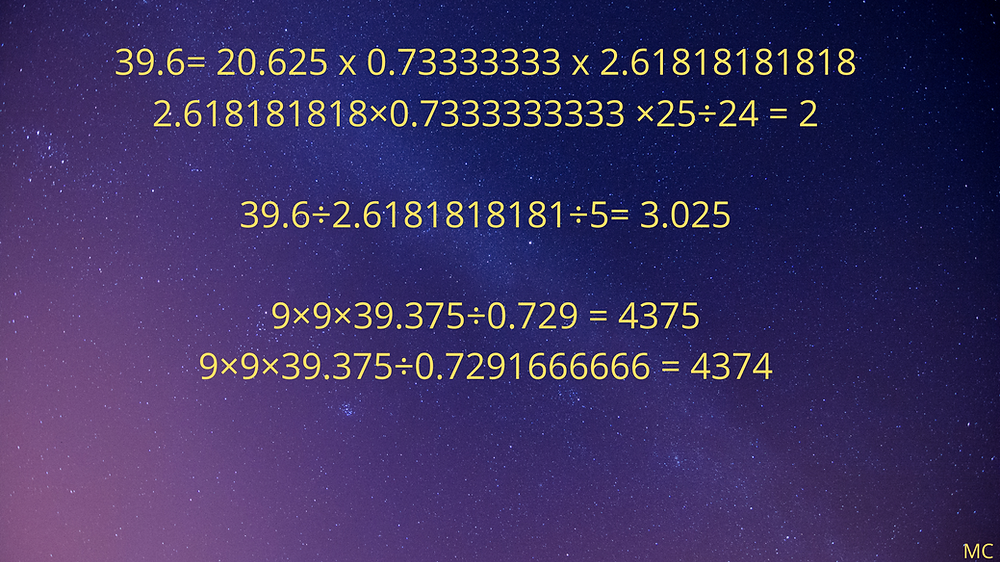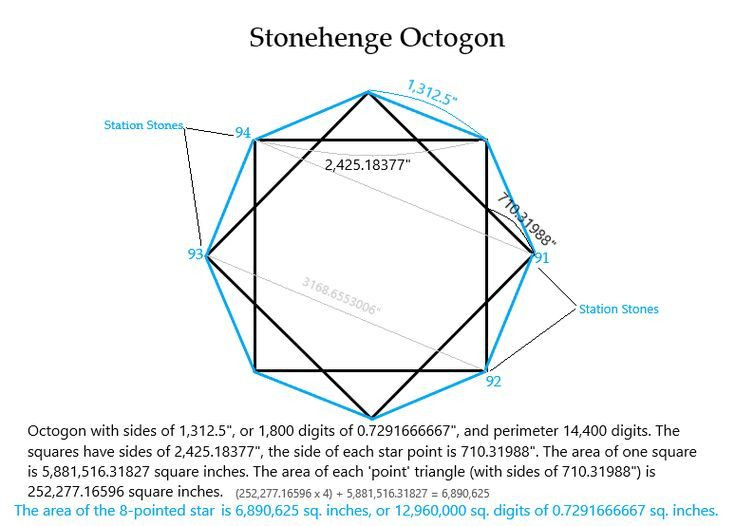Search

# 34. More Metrology and Geometry Connections

Updated: Feb 23

Many thanks to David Kenworthy for his fantastic video presentations on YouTube, and many thanks for all his encouragement, organising the various units in question into tables, and for sharing his ideas.

Here are the most recent of his videos: The Great Giza Pyramid Proving Pi/6 = Cubit / Meter, The Giza Great Pyramid and Berriman's Summary of Linear Units,

I thought I'd put some more diagrams and images I've put together into another post, to show more connections between some of the numbers that come up in ancient metrology.

Starting with this one which is a screen shot from a video presentation of John Neal's. I like this because it shows a clear progression between units in terms of fractions. It should be said that this approach, and many of the values assigned to the units, are sometimes quite different from those of other researchers, but the inner logic of Neal's system works. Amazing research.Another researcher who's done some very inspiring work is Hugh Franklin. I came across him thanks to David Kenworthy. This is a diagram I made after being struck by one of his findings, in relation to the equatorial circumference. Hugh Franklin’s insight about the link between the value of the circumference in miles and pi should perhaps be more widely known. In his article “Earth, Pi, Miles and the Barleycorn”, see http://hew_frank.tripod.com/epmb2.htm , he points out that the number 24,902.31984, which is very close to the contemporary figure for the equatorial circumference of the earth in miles, is in fact √(π3 x 20,000,000). So if you have a circle with a circumference of 24,902.31984, and the diameter of this circle is also the diagonal of a square. The square will have a side of 24,902.31984 /(√2x π), and the area of this square will be 10,000,000 π. This square would be equal in area to a circle with a diameter of 10,000,000. This would suggest that the equatorial circumference of our planet, via the geometries of the circle and the square, gave rise to the mile itself, as a unit of measure. The circumference of the earth as a value in miles is equated to a square with an area of 10,000,000 π. It’s worth noting that in this model, actual pi is used, not an approximation such as 22/7.

So, approximately,

• one mile = earth’s equatorial circumference /( √(π3 x 20,000,000)

= √(10,000,000 π) x √2 x π /earth’s equatorial circumference

• and the equatorial circumference of the earth

= 365.242199 x 360,000 =131,487,191.64 feet, or 24,902.877 miles

• the meridian circumference of the earth

=1,575,000,000 inches for some, i.e 24,857.954545 = 25,000 x 175/176 miles

or = 24,883.2 miles

(and there are several other interesting variations of these dimensions too, such as 24,888.888 miles, 40,000 km, etc.)

Taking inspiration from Robin Heath's work in The Lost Science of Measuring the Earth, you could say:

• One mile, in feet, can be defined as 70,000 lunations / (36 x the difference in days between solar and lunar years).

• Consequently, earth’s equatorial circumference in feet

= 70,000 lunations x (√(π3 x 20,000,000) / ( 36 x the difference in days between solar and lunar years)

= √(10,000,000 π) x √2 x π x 5,280

• and the equatorial circumference of the earth in miles

= 365.242199 x 1,296 x the difference in days between solar and lunar years / 7 lunations

the meridian circumference of the earth

= 1752 x 1,000,000 lunations / ( 3 x the difference in days between solar and lunar years x 176) inches

This means that the foot and the mile may be derived from the relationship between the solar year and twelve lunar months. (The 10.87512 is the difference in days between the solar and lunar years, and the 29.5306 is the number of days in a lunation, or lunar month. The length of a lunation has gradually decreased over the last few millennia, and the length of a solar years has increased slightly, so this 10.87512 number may not correspond to the number used long ago by astronomers.)The Earth's meridian circumference is always a source of great interest: are the most important units that makeup systems of measurement derived from it, and what is the total exact length that was used? Jim Alison's work in reconciling units of historical metrology to the 0.7291666667" digit, which is the division of the 1,575,000,000 inch meridian circumference by 2,160,000,000, is brilliant. See here.

Below is a chart showing some estimates for the meridian circumference of our planet that may have been in use, and how they relate to each other.To go back to the astronomical connections between units of measure, how exactly do the numbers reflect the cycles of the sun, moon, and other heavenly bodies? Here I try and look at some of the main numbers that crop up so often and how they express astronomical cycles.There are many geometric considerations to take into account also, and looking at units in terms of simple squares and circles offers many possibilities of creating meaning. The more complicated shapes also yield interesting things, in two and three dimensions.At Stonehenge, I was surprised to find a very nice number of square digits in the octogon created by the Station Stone Rectangle:Finally, why do the inch and the modern metre seem to embody a solar-lunar relationship? The modern inch has been redefined according to the metre. Why? Why is this relationship so important? Why was the metre not defined in relation to the inch? Especially when the 1,575,000,000 inches expressed in the 24,857.95454545 mile meridian circumference (equivalent to 40,000,000 metres of 39.375") are closer to the actual size of the planet than 40,000,000 modern metres.